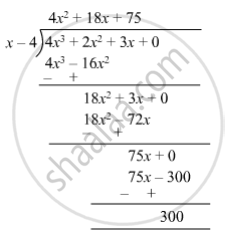Advertisement Remove all ads

# Divide and Write the Quotient and the Remainder. (3x + 2x2 + 4x3) ÷ (X − 4) - Mathematics

Sum

Divide and write the quotient and the remainder.

(3x + 2x2 + 4x3) ÷ (x − 4)

Advertisement Remove all ads

#### Solution

(3x + 2x2 + 4x3) ÷ (x − 4)So, quotient = $4 x^2 + 18x + 75$  and remainder = 300.

Concept: Divide a Polynomial by a Binomial
Is there an error in this question or solution?
Advertisement Remove all ads

#### APPEARS IN

Balbharati Mathematics 8th Standard Maharashtra State Board
Chapter 10 Division of Polynomials
Practice Set 10.2 | Q 3 | Page 66
Advertisement Remove all ads
Advertisement Remove all ads
Share
Notifications

View all notifications

Forgot password?